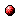# The extended linear complementarity problem and its applications in the analysis and control of discrete event systems and hybrid systems

Reference:
B. De Schutter and B. De Moor, "The extended linear complementarity problem and its applications in the analysis and control of discrete event systems and hybrid systems," Proceedings of the IEEE Singapore International Symposium on Control Theory and Applications (SISCTA'97), Singapore, pp. 394-398, July 1997.

Abstract:
In this paper we first present a mathematical programming problem, the Extended Linear Complementarity Problem (ELCP). Next we discuss how the ELCP can be used to solve some basic problems in the system theory for a class of discrete event systems that can be modeled using the max-plus algebra, which has maximization and addition as basic operations. Finally we show that the ELCP also appears in the analysis of certain classes of hybrid systems.Corresponding technical report: pdf file (137 KB)
Note: More information on the pdf file format mentioned above can be found here.

Bibtex entry:

@inproceedings{DeSDeM:97-34,
author={B. {D}e Schutter and B. {D}e Moor},
title={The extended linear complementarity problem and its applications in the analysis and control of discrete event systems and hybrid systems},
booktitle={Proceedings of the IEEE Singapore International Symposium on Control Theory and Applications (SISCTA'97)},